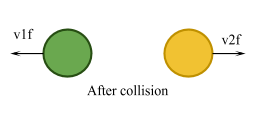# Newton’s Law of Restitution

Contents

## Summary

• Newton’s law of restitution states that when two bodies collide, the speed with which they move after the collision actually depends on the material from which they are made.
• The Coefficient of Restitution (e) tells us about the bounciness, or elasticity, of the collision.
• When e = 1, no kinetic energy is lost
• When e = 0, maximum kinetic energy is lost$e\quad =\quad \frac { relative\quad speed\quad after\quad collision }{ relative\quad speed\quad before\quad collision }$

Newton’s law of restitution states that when two bodies collide, the speed with which they move after the collision actually depends on the material from which they are made.

Let’s assume that when two balls collide, their velocity after the collision is dependent on the material from which they are made as shown in Fig 1 below.#### The coefficient of restitution

Now that you have understood the basic concept behind Newton’s Law of restitution, let’s move on to study what the coefficient of restitution is.

The Coefficient of Restitution (e) is a variable number with no units, with limits from zero to one.

Its value basically tells us about the bounciness, or elasticity, of the collision. A collision between two particles where no overall kinetic energy is lost is called a perfectly elastic collision, and these collisions are the most bouncy ones. They have a coefficient of restitution of e = 1.

On the other hand, a collision where the maximum kinetic energy is lost is called a perfectly inelastic collision, and this is where the two objects stick together and continue to move together after the collision. They are not bouncy at all. They have a coefficient of restitution of e = 0.

The main formula to work out the coefficient of restitution is:$e\quad =\quad \frac { relative\quad speed\quad after\quad collision }{ relative\quad speed\quad before\quad collision }$

Or it can be written as:$e\quad =\quad \frac { { V }_{ 2f }\quad -\quad { V }_{ 1f } }{ { V }_{ 2i }\quad -\quad { V }_{ 1i } }$

#### Example #1

Q. A 5 kg mass moving at 6$m{ s }^{ -1 }$  makes a head-on collision with a 4 kg mass travelling at 3$m{ s }^{ -1 }$ .
Assuming that there are no external forces acting on the system, what are the velocities of the two masses after impact? (assume coefficient of restitution e = 0.5 )

Solution:

From the question we can extract the following data:${ u }_{ A }\quad =\quad initial\quad speed\quad of\quad 5kg\quad mass\quad (mass\quad A)\quad =\quad 6\quad m{ s }^{ -1 }$${ u }_{ B }\quad =\quad initial\quad speed\quad of\quad 4kg\quad mass\quad (mass\quad B)\quad =\quad 3\quad m{ s }^{ -1 }$${ m }_{ A }\quad =\quad 5\quad kg$${ m }_{ B }\quad =\quad 4\quad kg$${ v }_{ A }\quad =\quad final\quad speed\quad of\quad mass\quad A$${ v }_{ B }\quad =\quad final\quad speed\quad of\quad mass\quad B$

We know that momentum before the collision equals momentum after
hence:${ m }_{ A }{ u }_{ A }\quad +\quad { m }_{ B }{ u }_{ B }\quad =\quad { m }_{ A }{ v }_{ A }\quad +\quad { m }_{ B }{ v }_{ B }$

also$e\quad =\quad \frac { { V }_{ B }\quad -\quad { V }_{ A } }{ { U }_{ B }\quad -\quad { U }_{ A } }$

substituting for$e,\quad { m }_{ A },\quad { u }_{ A },\quad { m }_{ B },\quad u_{ B }$

two simultaneous equations are formed from the conservation of momentum:$5\quad \times \quad 0.6\quad +\quad 4(-3)\quad =\quad 5{ v }_{ A }\quad +\quad 4{ v }_{ B }$$3\quad -\quad 12\quad =\quad 5{ v }_{ A }\quad +\quad 4{ v }_{ B }$$-9\quad =\quad 5{ v }_{ A }\quad +\quad 4{ v }_{ B }$$5{ v }_{ A }\quad +\quad 4{ v }_{ B }\quad =\quad -9\quad \quad \quad \quad \Rightarrow \quad (i)$

from the coefficient of restitution expression:$0.5\quad =\quad \frac { { V }_{ B }\quad -\quad { V }_{ A } }{ { U }_{ B }\quad -\quad { U }_{ A } }$$0.5({ u }_{ A }\quad -\quad { u }_{ B })\quad =\quad { v }_{ B }\quad -\quad { v }_{ A }$$(0.5\quad \times \quad 6)\quad -\quad (0.5(-3))\quad =\quad { v }_{ B }\quad -\quad { v }_{ A }$$3\quad +\quad 1.5\quad =\quad { v }_{ B }\quad -\quad { v }_{ A }$$4.5\quad =\quad { v }_{ B }\quad -\quad { v }_{ A }$${ v }_{ B }\quad -\quad { v }_{ A }\quad =\quad 4.5\quad \quad \quad \quad \Rightarrow \quad (ii)$

Finally we multiply equation (ii) by 5 and adding:$5{ v }_{ A }\quad +\quad 4{ v }_{ B }\quad =\quad -9$$-5{ v }_{ A }\quad +\quad 5{ v }_{ B }\quad =\quad 22.5$$9{ v }_{ B }\quad =\quad 13.5$${ v }_{ B }\quad =\quad 1.5\quad m{ s }^{ -1 }$    Ans

from equation (ii):$1.5\quad -\quad { v }_{ A }\quad =\quad 4.5$${ v }_{ A }\quad =\quad 1.5\quad -\quad 4.5\quad =\quad -3$${ v }_{ A }\quad =\quad -3\quad m{ s }^{ -1 }$    Ans

Therefore the velocities after collision of the 5 kg and 4 kg masses are -3$m{ s }^{ -1 }$ and 1.5$m{ s }^{ -1 }$,  respectively.